# Class A , Class B & Class C Power Amplifier

## Class A , Class B & Class C Power Amplifiers Classification

We have already discussed about audio power amplifiers in our previous tutorial. In this post we are going to discuss about different types of power amplifiers.

Power amplifiers are classified according to their mode of operation i.e the portion of the input cycle during which the collector current is expected to flow. On this basis the power amplifiers are classified as :

1. Class A power amplifier
2. Class B power amplifier
3. Class C power amplifier

Let us discuss each type of power amplifier in detail.

### Class A Power Amplifier

If the collector current flows all at all times during the full cycle of the signal, the power amplifier is known as class A power amplifier.

To achieve this, the power amplifier must be biased in such a way that no part of the signal is cut off.

The circuit diagram of a class A power amplifier is shown in fig. below.In case of a direct-coupled class A power amplifier as shown above, the current flows through the collector resistive load causes large wastage of dc power in it. As a result this dc power dissipated in the load resistor does not contribute to the useful ac output power.

Hence, it is generally inadvisable to pass the current through the output device such as in a voice coil of a loudspeaker.

For these reasons an arrangement using a suitable transformer for coupling the load to the amplifier is usually employed, as shown above.

This arrangement also permits impedance matching.

In a power amplifier circuit shown R1 and R2 provide potential divider biasing and emitter resistor RE is meant for bias stabilization. The emitter bypass capacitor CE is meant for RE to prevent ac voltage. The input capacitor Cin couples ac signal voltage to the base of the transistor but blocks any dc from the previous stage. A step-down transformer of suitable turn ratio is provided to couple the high impedance collector circuit to low impedance load.

### Impedance Matching

The power transferred from the power amplifier to the load such as loudspeaker, will be maximum only if the amplifier output imped­ance equals the load impedance RL. This is in accord­ance with the maximum power transfer theorem.

If we were not able to achieve the above condition, lesser power will be transferred to the load RL, though the amplifier is capable of delivering more power, and rest of power developed would be lost in the active device.

Hence for transfer of maximum power from amplifier to the output device matching of amplifier output impedance with the impedance of output device is necessary.

This is accomplished by using a step-down transformer of suitable turn-ratio.

The operation of a class A power amplifier as a.c. load line is shown in fig. below.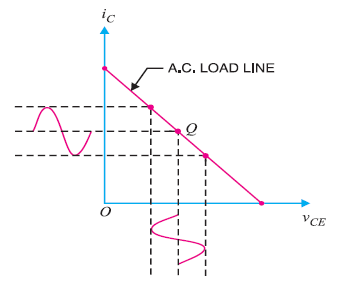The operating point Q is so selected that collector current flows at all times throughout the full cycle of the applied signal.

Since the output wave shape is exactly similar to the input wave shape, hence, such amplifiers have least distortion.

The only disadvantage of class A power amplifier is the low output power and low collector efficiency.

### Class B Power Amplifier

If the collector current flows only during the positive half-cycle of the input signal, it is called as class B power amplifier.

In class B power amplifier operation, the transistor is so adjusted that  zero signal collector current is zero i.e. no biasing circuit i needed at all.

During the positive half-cycle of the signal,  the input circuit is forward biased and hence collector current flows.

During the negative half-cycle, the input circuit is reverse biased and no collector current flows.

The operation of class B power amplifier in terms of  a.c. load line is shown in fig. below.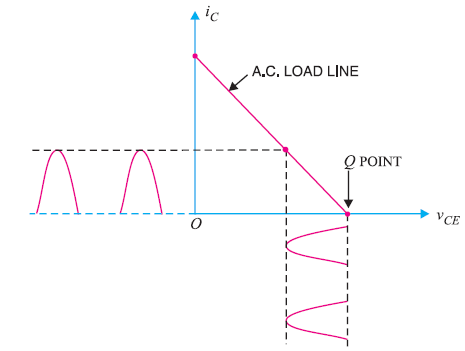As you can see, the operating point Q is located at collector cut off voltage.

In class B amplifier, the negative half-cycle of the signal is cut off and hence severe distortion occurs. However, it provides higher power output and hence collector efficiency.

These amplifiers are mostly used for power amplification in push-pull arrangement. In such arrangement, two transistors are used in class B operation. One transistor amplifies the positive half cycle of the signal and the other one amplifies the negative half-cycle of the signal.

### Class C Power Amplifier

If the collector current flows for less than half-cycle of the input signal, it is called class C power amplifier.

In class C power amplifier, the base is  negatively biased, so that collector current does not flow just when the positive half-cycle of the signal starts.

Such amplifiers are never used for power amplification but as tuned amplifier i.e. to amplify a narrow band of frequencies near the resonant frequency.

### Performance Quantities of Power Amplifier

The performance quantities or criteria for a power amplifier are:

1. Collector Efficiency
2. Distortion
3. Power Dissipation Capability

#### (i) Collector Efficiency

The main criterion for a power amplifier is not the power gain but the maximum a.c. power output.

An amplifier converts d.c. power from supply into a.c. power output. Hence, the effectiveness of a power amplifier is measured in terms of its ability to convert d.c. power from supply to a.c. output power. This is called as collector efficiency.

The collector efficiency is defined as the ratio of output power to the zero signal  power or d.c. power supplied by the battery.

#### Expression for collector efficiency

Collector efficiency,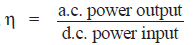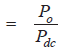Where,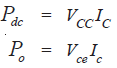Where Vce is the r.m.s. value of signal output voltage and Ic is the r.m.s. value of output signal current.

In terms of peak-to-peak values, the a.c. power output can be expressed as :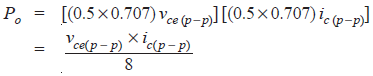#### (ii) Distortion

The change of output wave shape from the input wave shape of an amplifier is known as distortion.

Since , the transistor is a non-linear device, the output is not exactly like the input signal applied to it. Which means distortion occurs.

#### (iii) Power  Dissipation Capability

The ability of a power transistor to dissipate heat is known as power dissipation capability.

As we know , a power transistor handles large currents and hence, heats up during operation. Since, any temperature change influences the operation of transistor, therefore, the transistor must dissipate this heat to its surroundings.

To achieve this, normally a heat sink is attached to a power transistor case. The increased surface area allows heat to escape easily and keeps the case temperature of the transistor within permissible limit.